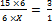## Ratio and Proportion For SBI PO : Set – 04

1) A man purchases some orange at the rate of 3 for Rs. 40 and the same quantity at 5 for Rs.60. If he sells all the oranges at the rate of 3 for Rs.50, find his gain or loss percent (to the nearest integer)

a) 34% loss

b) 31.5% loss

c) 31.5% profit

d) 34% profit

e) 35% Profit

(c)

Man purchased 15 oranges of each type

C.P of 1st type={40/3}*15=200

C.P of 2nd type={60/5}*15=180

Total cost price=(200+180)=380

Total selling price={50/3}*30=500

Profit percentage=(500-300)/380*100

=31.5%

2) Harsh has 50 paise, 25 paise and 10 paise coins in the ratio of 5 : 9 : 4 amounting to 412. Find the number of 10 paise coins.

a) 720

b) 240

c) 320

d) 400

e) 420

(c)

let ratio be x

Number of coins be 5x; 9x;4x respectively

(0.50)5x+(0.25)9x+(0.10)4x=412

25x+2.25x+0.4x=412

5.15x=412

X=80

4x=320

3) In a village 75% of the populations are literate of which the men and women are in the ratio 8:7 respectively. If the number of literate women is 70000. What is the total population of the village?

a) 1.5lacs

b) 2lacs

c) 70,000

d) 80,000

e) 50,000

b)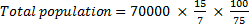= 2 lakhs

4) When a number is added to another number the total becomes 150 percent of the second number what is the ratio between the first and the second number?

a) 3:4

b) 2:1

c) 1:2

d) 4:3

e) 3:2

c)

x+y=150/100y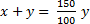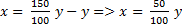x/y=1/2  = x:y = 1:2

5) If two numbers are in the ratio 7:12 and their least common multiple is 336, what is the sum of the numbers?

a) 57

b) 67

c) 75

d) 76

e) 78

d)

Two numbers are in the ratio 7:12

7x,12x; x must be the H. C. F

product of 2 numbers=H.C.F * L.C.M

7x*12x=336*x

x=4 ,

sum=19x=19*4=76

6) The ratio of the speed of two cars is 5:8. If the second car covers 300km in 3 hours find the speed of first car?

a) 65.5kmph

b) 62.5kmph

c) 70kmph

d) 72.5kmph

e) None of these

(b)

C1       C2

5x:     8x

C2=300/3=100km/hr

8x = 652.5 km/hr

7) The ratio of the number of students studying in schools A, B and C is 5 : 8 : 4 respectively. If the number of students studying in each of the schools in increased by 20%, 25% and 30% respectively, what will be the new respective ratio of the students in school A, B, and C?

a) 13 : 25 : 15

b) 20 : 15 : 13

c) 15 : 25 : 13

d) 25:15:30

e) None of these

(c)

A        : B      : C

50      : 8      : 4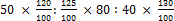600: 1000: 520

15: 25: 13

8) A man spends 80% of his monthly salary on rent, EMI and miscellaneous expenses in the ratio of 3:1:4 . If he spends a total of 16000rs on rent and EMI together, how much is his monthly salary?

a) 44000

b) 35000

c) 40000

d) 48000

e) 50000

(c)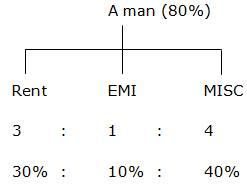Rent + EMI = 40%

40%    = 16000

100%  = x

x= 40000

10) Rahul and Gaurav started a business in the ratio of 15:6 and their profit were in the ratio of 3:1. If Rahul invested the money for 6 months, then for what time did Gaurav invest the money?

a) 3months

b) 5months

c) 6months

d) 7months

e) None of these